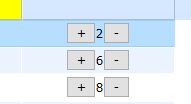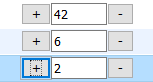Grid eXcell_button with 2 button inside cell

#1

Hi all,i want add increase and decrease method to button + and - for the centered number and i want the number keep editable

my code:

function eXcell_button(cell){ //the eXcell name is defined here
if (cell){ //the default pattern, just copy it
this.cell = cell;
this.grid = this.cell.parentNode.grid;
eXcell_ed.call(this); //uses methods of the “ed” type
}
this.setValue=function(val){
this.setCValue("<input type=‘button’ value=’+’>"+val+"<input type=‘button’ value=’-’>");
}
this.getValue=function(){
return this.cell.firstChild.value; // get button label
}
}
eXcell_button.prototype = new eXcell;

#2

Hi All,
I’m close to the goal , but i don’t increase or decrease on event,
i need helpmy new code :

function eXcell_button(cell){ //the eXcell name is defined here
if (cell){ //the default pattern, just copy it
this.cell = cell;
this.grid = this.cell.parentNode.grid;
// eXcell_ed.call(this); //uses methods of the “ed” type
}

this.edit=function(){
this.val = this.getValue(); // save current value
this.val = this.cell.childNodes.value;
this.cell.childNodes.value=parseInt(this.val); // set the first part of data
}

this.setValue=function(val){

console.log(“ici val =”+ val);
this.setCValue("<input type=‘button’ value=’+’>"+"<input type=‘text’ style=‘width:50px;’ value=’"+val+"’>"+"<input type=‘button’ value=’-’>");

this.cell.childNodes.onclick=function(e){
(e||event).cancelBubble=true;
console.log(“button 1=”+ val);
}

this.cell.childNodes.onclick=function(e){
(e||event).cancelBubble=true;
console.log(“button 2=” + val );
}
}
this.getValue=function() {
///return this.cell.firstChild.value; // get button label
return this.cell.childNodes.value;
}

this.detach=function(){
return this.val!=this.cell.childNodes.value;
}

}
eXcell_button.prototype = new eXcell;

#3

Please, try to use the following solution:
http://snippet.dhtmlx.com/5/dbb003c42